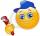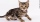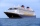# Fraction calculator

The calculator performs basic and advanced operations with fractions, expressions with fractions combined with integers, decimals, and mixed numbers. It also shows detailed step-by-step information about the fraction calculation procedure. Solve problems with two, three, or more fractions and numbers in one expression.

## Result:

### 2/3 * 1/2 = 1/3 ≅ 0.3333333

Spelled result in words is one third.

### How do you solve fractions step by step?

1. Multiple: 2/3 * 1/2 = 2 · 1/3 · 2 = 2/6 = 1 · 2/3 · 2 = 1/3
Multiply both numerators and denominators. Result fraction keep to lowest possible denominator GCD(2, 6) = 2. In the next intermediate step, , cancel by a common factor of 2 gives 1/3.
In words - two thirds multiplied by one half = one third.

#### Rules for expressions with fractions:

Fractions - use the slash “/” between the numerator and denominator, i.e., for five-hundredths, enter 5/100. If you are using mixed numbers, be sure to leave a single space between the whole and fraction part.
The slash separates the numerator (number above a fraction line) and denominator (number below).

Mixed numerals (mixed fractions or mixed numbers) write as non-zero integer separated by one space and fraction i.e., 1 2/3 (having the same sign). An example of a negative mixed fraction: -5 1/2.
Because slash is both signs for fraction line and division, we recommended use colon (:) as the operator of division fractions i.e., 1/2 : 3.

Decimals (decimal numbers) enter with a decimal point . and they are automatically converted to fractions - i.e. 1.45.

The colon : and slash / is the symbol of division. Can be used to divide mixed numbers 1 2/3 : 4 3/8 or can be used for write complex fractions i.e. 1/2 : 1/3.
An asterisk * or × is the symbol for multiplication.
Plus + is addition, minus sign - is subtraction and ()[] is mathematical parentheses.
The exponentiation/power symbol is ^ - for example: (7/8-4/5)^2 = (7/8-4/5)2

#### Examples:

subtracting fractions: 2/3 - 1/2
multiplying fractions: 7/8 * 3/9
dividing Fractions: 1/2 : 3/4
exponentiation of fraction: 3/5^3
fractional exponents: 16 ^ 1/2
adding fractions and mixed numbers: 8/5 + 6 2/7
dividing integer and fraction: 5 ÷ 1/2
complex fractions: 5/8 : 2 2/3
decimal to fraction: 0.625
Fraction to Decimal: 1/4
Fraction to Percent: 1/8 %
comparing fractions: 1/4 2/3
multiplying a fraction by a whole number: 6 * 3/4
square root of a fraction: sqrt(1/16)
reducing or simplifying the fraction (simplification) - dividing the numerator and denominator of a fraction by the same non-zero number - equivalent fraction: 4/22
expression with brackets: 1/3 * (1/2 - 3 3/8)
compound fraction: 3/4 of 5/7
fractions multiple: 2/3 of 3/5
divide to find the quotient: 3/5 ÷ 2/3

The calculator follows well-known rules for order of operations. The most common mnemonics for remembering this order of operations are:
PEMDAS - Parentheses, Exponents, Multiplication, Division, Addition, Subtraction.
BEDMAS - Brackets, Exponents, Division, Multiplication, Addition, Subtraction
BODMAS - Brackets, Of or Order, Division, Multiplication, Addition, Subtraction.
GEMDAS - Grouping Symbols - brackets (){}, Exponents, Multiplication, Division, Addition, Subtraction.
Be careful, always do multiplication and division before addition and subtraction. Some operators (+ and -) and (* and /) has the same priority and then must evaluate from left to right.

## Fractions in word problems:

• Equivalent fractionsAre these two fractions equivalent -4/9 and 11/15?
• WomenIn a company of 1050 employees are 2/3 women. How many women work in the company?
• SomeoneSomeone ate 1/10 of a cake, leaving only 9/10. If you eat 2/3 of the cake that is left, how much of a whole cake will you have eaten?
• One third 2One third of all students in class live in a house. If here are 42 students in a class, how many of them live in house?
• SarahSarah has a photography blog. 3/7 of her photos are of nature, 1/4 of the rest are of her friends. What fraction of all of Sarah's photos is of her friends?
• RickyRicky painted 3/5 of the side of the garage. When he repainted ½ of this part, what part of the side of the garage did he painted twice?
• Fraction expressionWhich expression is equivalent to : minus 9 minus left parenthesis minus 4 start fraction 1 divided by 3 end fraction right parenthesis
• Recipe 2Anna's making muffins! These ingredients make 12 muffins: 3/4 cup of flour 1/2 cup of sugar 1/4 teaspoon salt 1 cup of milk 1/3 teaspoon cinnamon 7 tablespoons butter 2 teaspoons baking powder 1 egg 2 tablespoons brown sugar Anna has a 8 muffin tray. What
• The kennel20 dogs. Ratio dogs to cats is 3:2  how many cats?
• Chocolate chipOut of 28 cookies, 3/4 are chocolate chip. How many cookies are chocolate chip?
• Quotient 3If the quotient of 8/13 and 2 is subtracted from the product of 1 3/4 and 8/21, what is the difference?
• In centerWhat number lies in the center between a quarter of a fifth and a half of a third on the number axis?
• Crimson LynxCaptain Emily has a ship, the H. M. S Crimson Lynx. The ship is five furlongs from the dread pirate Umaima and her merciless band of thieves. If her ship hasn't already been hit, Captain Emily has probability 3/5 of hitting the pirate ship. If her ship ha